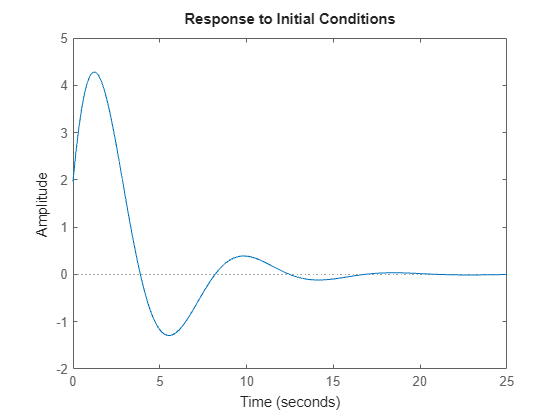Main Content

# initial

Initial condition response of state-space model

## Syntax

```initial(sys,x0) initial(sys,x0,Tfinal) initial(sys,x0,t) initial(sys1,sys2,...,sysN,x0) initial(sys1,sys2,...,sysN,x0,Tfinal) initial(sys1,sys2,...,sysN,x0,t) [y,t,x] = initial(sys,x0) [y,t,x] = initial(sys,x0,Tfinal) [y,t,x] = initial(sys,x0,t) ```

## Description

`initial(sys,x0)` calculates the unforced response of a state-space (`ss`) model `sys` with an initial condition on the states specified by the vector `x0`:

`$\begin{array}{cc}\stackrel{˙}{x}=Ax,& x\left(0\right)={x}_{0}\\ y=Cx& \end{array}$`

This function is applicable to either continuous- or discrete-time models. When invoked without output arguments, `initial` plots the initial condition response on the screen.

`initial(sys,x0,Tfinal)` simulates the response from `t = 0` to the final time `t = Tfinal`. Express `Tfinal` in the system time units, specified in the `TimeUnit` property of `sys`. For discrete-time systems with unspecified sample time (```Ts = -1```), `initial` interprets `Tfinal` as the number of sampling periods to simulate.

`initial(sys,x0,t)` uses the user-supplied time vector `t` for simulation. Express `t` in the system time units, specified in the `TimeUnit` property of `sys`. For discrete-time models, `t` should be of the form `0:Ts:Tf`, where `Ts` is the sample time. For continuous-time models, `t` should be of the form `0:dt:Tf`, where `dt` becomes the sample time of a discrete approximation to the continuous system (see `impulse`).

To plot the initial condition responses of several LTI models on a single figure, use

`initial(sys1,sys2,...,sysN,x0)`

`initial(sys1,sys2,...,sysN,x0,Tfinal)`

`initial(sys1,sys2,...,sysN,x0,t)`

(see `impulse` for details).

When invoked with output arguments,

`[y,t,x] = initial(sys,x0)`

`[y,t,x] = initial(sys,x0,Tfinal)`

`[y,t,x] = initial(sys,x0,t)`

return the output response `y`, the time vector `t` used for simulation, and the state trajectories `x`. No plot is drawn on the screen. The array `y` has as many rows as time samples (length of `t`) and as many columns as outputs. Similarly, `x` has `length(t)` rows and as many columns as states.

## Examples

collapse all

Plot the response of the following state-space model:

`$\begin{array}{rcl}\left[\begin{array}{l}{\underset{}{\overset{˙}{x}}}_{1}\\ {\underset{}{\overset{˙}{x}}}_{2}\end{array}\right]& =& \left[\begin{array}{cc}-0.5572& -0.7814\\ 0.7814& 0\end{array}\right]\left[\begin{array}{l}{x}_{1}\\ {x}_{2}\end{array}\right]\\ y& =& \left[\begin{array}{cc}1.9691& 6.4493\end{array}\right]\left[\begin{array}{l}{x}_{1}\\ {x}_{2}\end{array}\right].\end{array}$`

Take the following initial condition:

`$x\left(0\right)=\left[\begin{array}{l}1\\ 0\end{array}\right].$`

```a = [-0.5572, -0.7814; 0.7814, 0]; c = [1.9691 6.4493]; x0 = [1 ; 0]; sys = ss(a,[],c,[]); initial(sys,x0)```## Tips

You can change the properties of your plot, for example the units. For information on the ways to change properties of your plots, see Ways to Customize Plots.

## See Also

Introduced before R2006a

## Support

#### Learn how to automatically tune PID controller gains

Download code examples